1. 清华大学 电机工程与应用电子技术系, 电力系统及发电设备安全控制和仿真国家重点实验室, 北京 100084;
2. 中车株洲电力机车研究所有限公司, 株洲 412000;
3. 浙江大学 电气工程学院, 杭州 310027

Energy balancing control for locomotive converter based on a discrete state event driven method
JU Jiahe1, ZHAO Zhengming1, SHI Bochen1, ZHU Yicheng1, YU Zhujun1, LUO Yunfei2, ZHANG Zhixue2, HU Sideng3, HE Xiangning3
1. State Key Laboratory of Control and Simulation of Power Systems and Generation Equipment, Department of Electrical Engineering, Tsinghua University, Beijing 100084, China;
2. CRRC Zhuzhou Institute Co., Ltd., Zhuzhou 412000, China;
3. College of Electrical Engineering, Zhejiang University, Hangzhou 310027, China
Abstract: Traction-oriented power electronic converters that are widely used in electric locomotives need to be very reliable and to avoid over-voltage problems caused by device stresses, so such devices need very stable DC bus voltages. Since the traction converter parameters are functions of the locomotive operating conditions, the single-phase rectifier control strategy must be very reliable to ensure the DC bus voltage stability. This paper presents an energy balancing control strategy for a single-phase rectifier for a traction converter which improves the converter dynamics. The control strategy needs to be tested for many different parameters and working conditions. A discrete state event driven (DSED) simulation method was used here in a numerical simulation platform for the traction converter for efficient, accurate multiple time scale (system level dynamic processes and device level transient processes) simulations. The traction converter simulation platform was used to compare the single-phase energy balance control with traditional PI control. The energy balance control is fast and effectively suppresses the DC bus fluctuations for all kinds of dynamic processes. Combining the energy balance control with the bus structure optimization also significantly reduces the turn-off electric force and allowance for the device.
Key words: locomotive converter    energy balance control    discrete state event driven (DSED)    power electronics simulation

1) 提高电力电子变换器的效率和功率密度，主要发展集成技术和冷却技术;

2) 实施精确控制，应用高性能的闭环控制;

3) 保证可靠运行，采用冗余控制和能量综合管理技术。

DSED仿真体系[12-16]基于对电力电子系统离散与连续的辩证认识，创新性地提出了瞬态模型、数值算法、仿真机制、模块解耦等方法，使得电力电子电路的仿真速度得到了极大的提升，是能够完成瞬态仿真且准确高效的仿真工具。有关DSED的算法细节可以参见文[12-16]，算法应用参见文中的大规模电能路由器算例。本文将DSED仿真机制应用于牵引变流器的仿真中，通过对比DSED与目前专业的电力电子仿真软件PLECS®，研究、评估和展示了DSED在电力电子与电机集成系统仿真方面的准确性与高效性。

1 DSED仿真方法

2 DSED仿真包含异步电机的牵引变流器 2.1 DSED仿真非线性系统

DSED仿真方法并不局限于文[12-17]中所提的仅包含线性元件的系统，同时也适用于包含电机类元件的非线性系统，如式(1)非线性状态方程所示。DSED利用其仿真机制对电机的非线性状态方程解算，能够高效准确地得到仿真结果。下文将以一个具体实例来说明DSED仿真非线性系统的准确性与高效性，为后续控制策略的研究奠定基础。

 $\left\{ {\begin{array}{*{20}{c}} {\frac{{{\rm{d}}{i_{{{\rm{s}}_d}}}}}{{{\rm{d}}t}} = \frac{{{L_{\rm{m}}}}}{{\sigma {L_{\rm{s}}}{L_{\rm{r}}}{T_{\rm{r}}}}}{\mathit{\Psi }_{{{\rm{r}}_d}}} + \frac{{{L_{\rm{m}}}}}{{\sigma {L_{\rm{s}}}{L_{\rm{r}}}}}{\omega _2}{\mathit{\Psi }_{{{\rm{r}}_q}}} - }\\ {\frac{{{r_{\rm{s}}}L_{\rm{r}}^2 + {r_{\rm{r}}}L_{\rm{s}}^2}}{{\sigma {L_{\rm{s}}}L_{\rm{r}}^2}}{i_{{{\rm{s}}_d}}} + \frac{{{u_{{{\rm{s}}_d}}}}}{{\sigma {L_{\rm{s}}}}}, }\\ {\frac{{{\rm{d}}{i_{{{\rm{s}}_\mathit{q}}}}}}{{{\rm{d}}t}} = \frac{{{L_{\rm{m}}}}}{{\sigma {L_{\rm{s}}}{L_{\rm{r}}}{T_{\rm{r}}}}}{\mathit{\Psi }_{{r_{\rm{q}}}}} - \frac{{{L_{\rm{m}}}}}{{\sigma {L_{\rm{s}}}{L_{\rm{r}}}}}{\omega _2}{\mathit{\Psi }_{{{\rm{r}}_d}}} - }\\ {\frac{{{r_{\rm{s}}}L_{\rm{r}}^2 + {r_r}L_{\rm{s}}^2}}{{\sigma {L_{\rm{s}}}L_{\rm{r}}^2}}{i_{{{\rm{s}}_q}}} + \frac{{{u_{{{\rm{s}}_q}}}}}{{\sigma {L_{\rm{s}}}}}, }\\ {\frac{{{\rm{d}}{\mathit{\Psi }_{{{\rm{r}}_d}}}}}{{{\rm{d}}t}} = - \frac{1}{{{T_{\rm{r}}}}}{\Psi _{{{\rm{r}}_d}}} - {\omega _2}{\mathit{\Psi }_{{{\rm{r}}_q}}} + \frac{{{L_{\rm{m}}}}}{{{T_{\rm{r}}}}}{i_{{{\rm{s}}_d}}}, }\\ {\frac{{{\rm{d}}{\mathit{\Psi }_{{{\rm{r}}_q}}}}}{{{\rm{d}}t}} = - \frac{1}{{{T_{\rm{r}}}}}{\Psi _{{{\rm{r}}_q}}} + {\omega _2}{\mathit{\Psi }_{{{\rm{r}}_d}}} + \frac{{{L_{\rm{m}}}}}{{{T_{\rm{r}}}}}{i_{{{\rm{s}}_q}}}, }\\ {\frac{{{\rm{d}}{\omega _2}}}{{{\rm{d}}t}} = - \frac{{p_0^2{L_{\rm{m}}}}}{{J{L_{\rm{r}}}}}\left( {{i_{{{\rm{s}}_q}}}{\Psi _{{{\rm{r}}_d}}} - {i_{{{\rm{s}}_d}}}{\Psi _{{{\rm{r}}_q}}}} \right) - \frac{{{p_0}}}{J}{T_{\rm{L}}}.} \end{array}} \right.$ (1)

2.2 DSED仿真包含异步电机模块的算例：某牵引变流器 2.2.1 仿真算例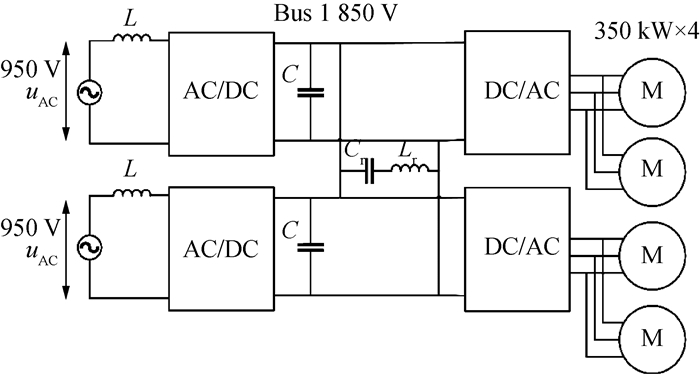图 1 牵引变流器简化拓扑

 参数名称 数值 输入交流电源有效值/V 950 直流母线电压/V 1 850 单个电机额定功率/kW 350 电机极数 4 电机额定转速/(r·min-1) 4 174 电机额定转矩/(N·m) 800

2.2.2 DSED与PLECS®仿真结果对比

DSED仿真牵引变流器带动电机启动到1 800 r/min的过程，与PLECS®启动0.1 s的过程波形对比可以得到图 2，具体的误差分析见表 2，仿真时长对比见表 3。相对误差的计算为

 \begin{aligned} \varepsilon_{i}=&\left|\frac{x_{\sin , i}-x_{\mathrm{ref}, i}}{x_{\mathrm{ref}, i}}\right| , \\ & \varepsilon=\sum\limits_{i=1}^{N} \frac{\varepsilon_{i}}{N} . \end{aligned} (2)
 \begin{aligned} \varepsilon=& \frac{\left\|x_{\mathrm{sim}}-x_{\mathrm{ref}}\right\|_{2}}{\left\|x_{\mathrm{ref}}\right\|_{2}}. \end{aligned} (3)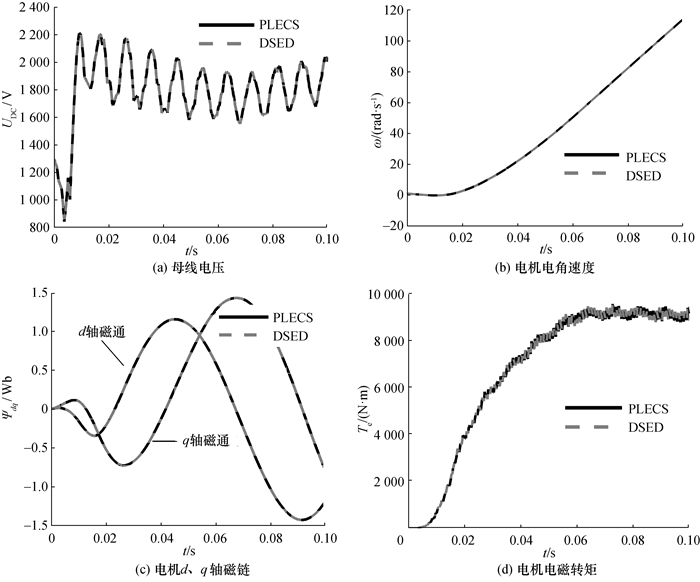图 2 启动过程仿真结果DSED与PLECS®对比

 软件 仿真场景 仿真设置 仿真耗时/s 相对误差电容电压/% 相对误差电机电角速度/% DSED 20 s电机启动过程理想开关模型 变步长算法最大步长1×10-3最大/最小阶数5/2绝对误差1×10-5 6.988 0.000 00571(式(3)) 0.000 000185(式(4)) 0.003 94(式(3))0.000 615(式(4)) PLECS© 20 s电机启动过程理想开关模型 变步长算法最大步长1×10-3DOPRI求解器绝对误差1×10-5 20.87 0.000 00600(式(3))0.000 00203(式(4)) 0.013 5(式(3))0.000 568(式(4))

 仿真时间过程/s DSED仿真时长/s PLECS®仿真时长/s 提速比例 20 6.988 20.87 1:3.0 120 24.758 126.26 1:5.1

2.2.3 DSED仿真结果与实验结果对比图 3 实验测试平台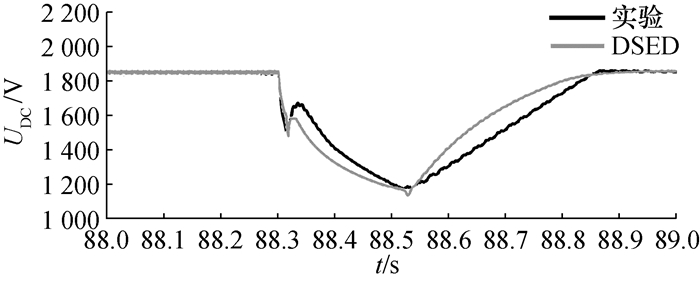图 4 DSED与实验对比母线电压跌落波形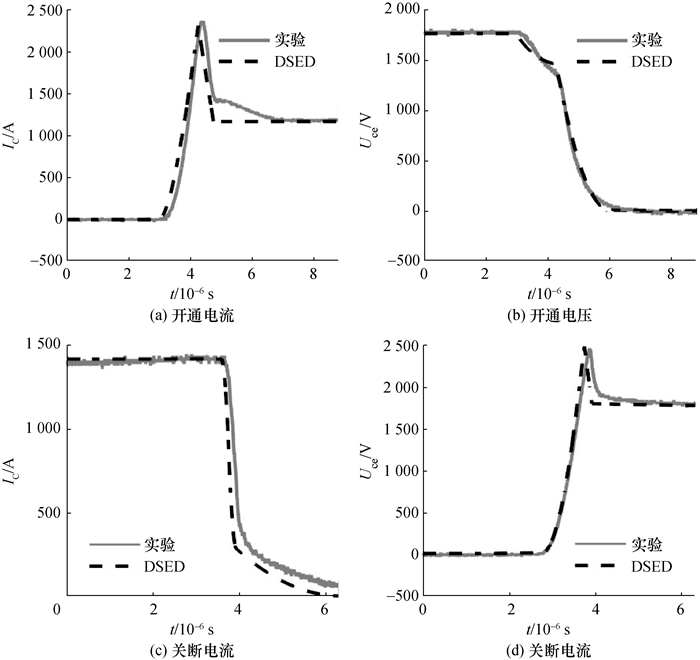图 5 DSED与实验对比双脉冲实验波形

3 基于DSED平台的单相整流器能量平衡控制

3.1 单相能量平衡控制算法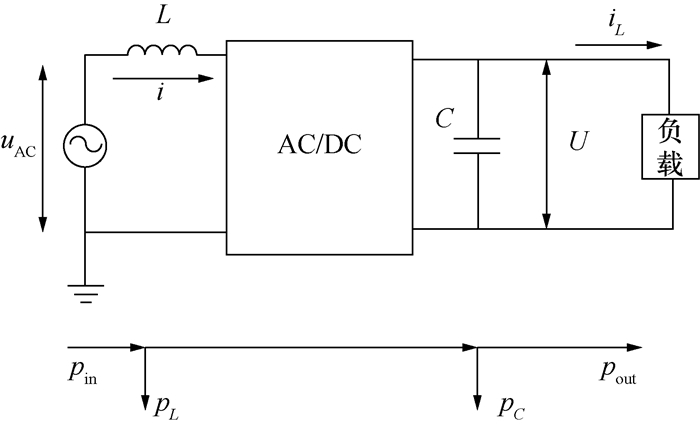图 6 单相整流器能量关系示意图

 $\begin{array}{*{20}{c}} {p_{{\rm{in }}}^*T = \frac{1}{2}L{i^{*2}} - \frac{1}{2}L{i^2} + \frac{1}{2}C{U^{*2}} - }\\ {\frac{1}{2}C{U^2} + {p_{{\rm{out }}}}T, } \end{array}$ (4)
 ${p_{{\rm{in }}}^*T = {i^*}{u_{{\rm{AC }}}}T, }$ (5)
 ${{p_{{\rm{out }}}}T = U{i_L}T.}$ (6)

 $i^{*} u_{\mathrm{AC}} \frac{T_{\mathrm{g}}}{2}=\frac{1}{2} C U^{* 2}-\frac{1}{2} C U^{2}+U i_{L} \frac{T_{\mathrm{g}}}{2}.$ (7)

 $f=\frac{1}{2 \pi \sqrt{L_{\mathrm{r}} C_{\mathrm{r}}}}=100.12 \mathrm{Hz}.$ (8)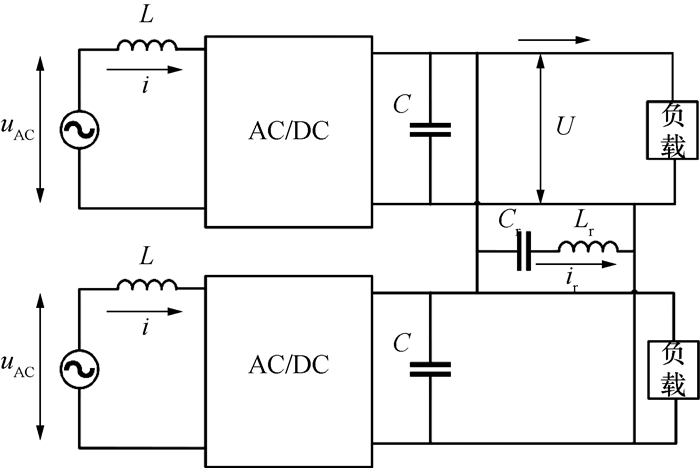图 7 并联单相整流器电路示意图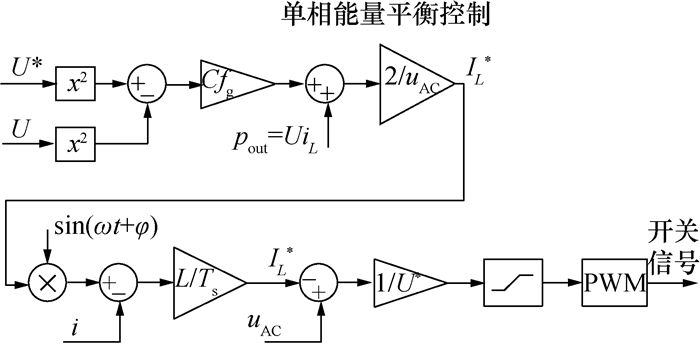图 8 单相能量平衡控制框图

3.2 DSED仿真平台下单相能量平衡控制的实现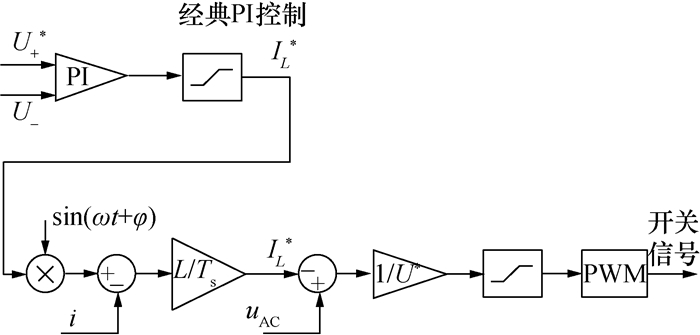图 9 经典PI控制框图

3.2.1 经典PI控制存在的问题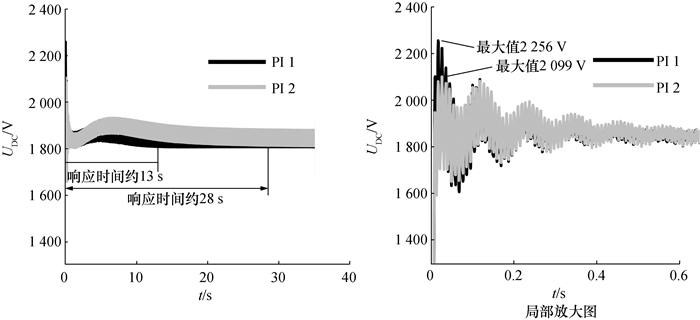图 10 经典PI控制下的启动过程中直流母线电压波形(2组参数对比)图 11 经典PI控制下的启动过程关断时刻的关断电压波形

3.2.2 单相能量平衡控制与经典PI控制对比

a) 负载变化。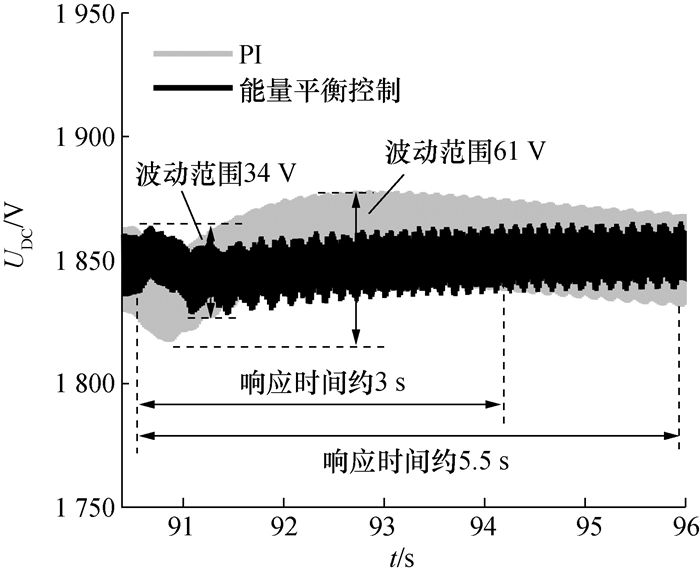图 12 经典PI控制与能量平衡控制对比—电机负载突变

b) 直流母线电压指令值变化。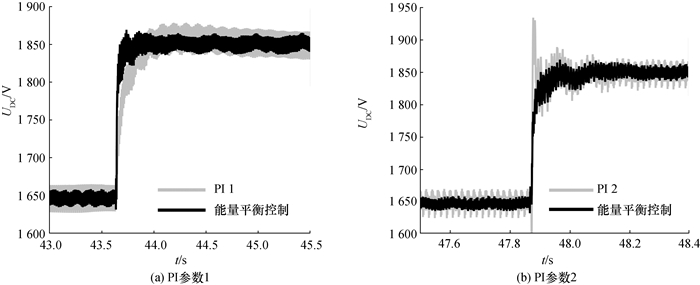图 13 经典PI控制与能量平衡控制对比—母线电压指令值变化

 指标 能量平衡控制 PI控制1 百分比/% PI控制2 百分比/% 超调(相对1 850 V)/V 19 28 — 85 77.6 响应时间/s 0.36 2.25 84.0 0.29 —

3.2.3 单相能量平衡控制及直流母排结构对开关瞬态过程的影响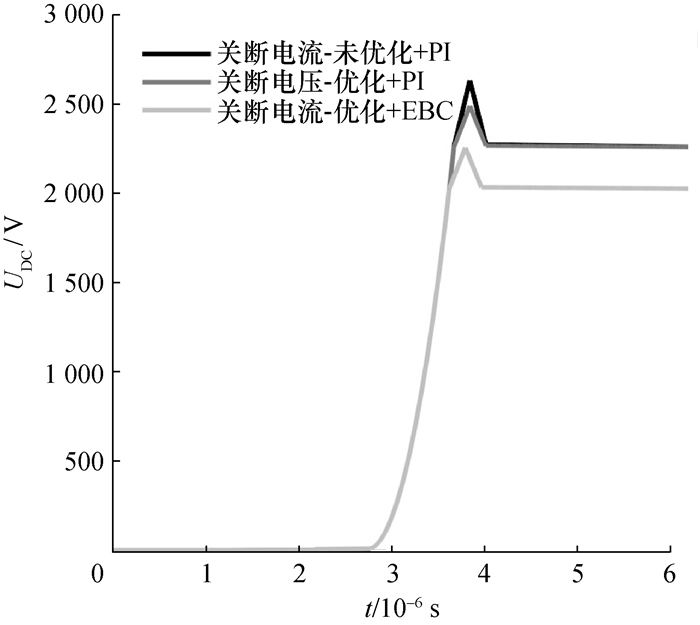图 14 不同控制与母排结构下的开关关断电压对比

 母排结构及控制方法 电压尖峰大小(相对稳态值)/V 电压最大值/V 常规母排结构+PI 364 2 620 模组电容连接母排结构+PI 229 2 485 模组电容连接母排结构+EBC 227 2 250

4 结论

  吴新红. 混合动力动车组牵引及辅助变流器系统分析[J]. 城市轨道交通研究, 2018, 21(11): 119-123. WU X H. Analysis of traction converter unit and auxiliary converter unit systems in hybrid EMU[J]. Urban Mass Transit, 2018, 21(11): 119-123. (in Chinese)  陈亚爱, 石永帅, 周京华, 等. 电力电子牵引变压器拓扑结构综述[J]. 电气传动, 2018, 48(10): 89-96. CHEN Y A, SHI Y S, ZHOU J H, et al. Overview of topological structure for power electronic traction transformer[J]. Electric Drive, 2018, 48(10): 89-96. (in Chinese)  谢宝成. 电力电子牵引变压器的工作原理及建模分析[J]. 自动化与仪器仪表, 2018(8): 134-136. XIE B C. Working principle and modeling analysis of power electronic traction transformer[J]. Automation & Instrumentation, 2018(8): 134-136. (in Chinese)  夏焰坤. 电力电子变压器的高速铁路同相供电系统及控制方法[J]. 电力系统及其自动化学报, 2019, 31(5): 98-102. XIA Y K. Cophase power supply system for high-speed railway based on power electronic transformer and its control method[J]. Proceedings of the CSU-EPSA, 2019, 31(5): 98-102. (in Chinese)  戈旺.基于电力电子变压器的新型牵引系统分析与控制[D].南昌: 华东交通大学, 2018. GE W. Analysis and control of new traction system based on power electronic transformer[D]. Nanchang: East China Jiaotong University, 2018. (in Chinese) http://cdmd.cnki.com.cn/Article/CDMD-10404-1018819121.htm  赵争鸣, 袁立强, 鲁挺, 等. 我国大容量电力电子技术与应用发展综述[J]. 电气工程学报, 2015, 10(4): 26-34. ZHAO Z M, YUAN L Q, LU T, et al. Overview of the developments on high power electronic technologies and applications in China[J]. Journal of Electrical Engineering, 2015, 10(4): 26-34. (in Chinese)  赵争鸣, 施博辰, 朱义诚. 对电力电子学的再认识——历史、现状及发展[J]. 电工技术学报, 2017, 32(12): 5-15. ZHAO Z M, SHI B C, ZHU Y C. Reconsideration on power electronics:the past, present and future[J]. Transactions of China Electrotechnical Society, 2017, 32(12): 5-15. (in Chinese)  赵争鸣, 袁立强, 鲁挺. 电力电子系统电磁瞬态过程[M]. 北京: 清华大学出版社, 2017. ZHAO Z M, YUAN L Q, LU T. The electromagnetic transient process of power electronic system[M]. Beijing: Tsinghua University Press, 2017. (in Chinese)  HE F B, ZHAO Z M, LU T, et al. Predictive DC voltage control for three-phase grid-connected PV inverters based on energy balance modeling[C]//The 2nd International Symposium on Power Electronics for Distributed Generation Systems. Hefei, China: IEEE, 2010: 516-519.  尹璐.基于能量平衡的四象限双PWM变频器控制策略研究[D].北京: 清华大学, 2013. YIN L. Research on control strategy of four quadrant dual PWM inverter based on energy balance control[D]. Beijing: Tsinghua University, 2013. (in Chinese)  贺凡波.大规模太阳能光伏并网发电系统电气设计与综合控制[D].北京: 清华大学, 2012. HE F B. Electrical design and integrated control of large-scale photovoltaic grid connected power generation system[D]. Beijing: Tsinghua University, 2012. (in Chinese)  SHI B C, ZHAO Z M, ZHU Y C. Piecewise analytical transient model for power switching device commutation unit[J]. IEEE Transactions on Power Electronics, 2018, 34(6): 5720-5736.  LI B Y, ZHAO Z M, YANG Y, et al. A novel simulation method for power electronics:Discrete state event driven method[J]. CES Transactions on Electrical Machines and Systems, 2017, 1(3): 273-282.  杨祎, 赵争鸣, 檀添, 等. 离散状态事件驱动仿真方法及自适应预估校正算法[J]. 电工技术学报, 2017, 32(12): 33-41. YANG Y, ZHAO Z M, TAN T, et al. Discrete state event driven method and self-adapted predictor-corrector algorithm[J]. Transactions of China Electrotechnical Society, 2017, 32(12): 33-41. (in Chinese)  李帛洋, 赵争鸣, 檀添, 等. 后向离散状态事件驱动电力电子仿真方法[J]. 电工技术学报, 2017, 32(12): 42-49. LI B Y, ZHAO Z M, TAN T, et al. A backword discrete state event driven simulation method for power electronics based on finite state machine[J]. Transactions of China Electrotechnical Society, 2017, 32(12): 42-49. (in Chinese)  ZHU Y C, ZHAO Z M, SHI B C, et al. Discrete state event-driven framework with a flexible adaptive algorithm for simulation of power electronic systems[J]. IEEE Transactions on Power Electronics, 2019, 34(12): 11692-11705.  施博辰, 赵争鸣, 朱义诚, 等. 离散状态事件驱动仿真方法在高压大容量电力电子变换系统中的应用[J]. 高电压技术, 2019, 45(7): 2053-2061. SHI B C, ZHAO Z M, ZHU Y C, et al. Application of discrete state event-driven simulation framework in high-voltage power electron-is hybrid systems[J]. High Voltage Engineering, 2019, 45(7): 2053-2061. (in Chinese)  赵争鸣, 袁立强. 电力电子与电机系统集成分析基础[M]. 北京: 机械工业出版社, 2009. ZHAO Z M, YUAN L Q. Lntegrated analysis of power electronics and motor drive system[M]. Beijing: Mechanical Industry Press, 2009. (in Chinese)